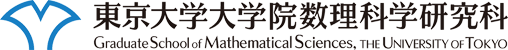このページに含まれる映像は自由に視聴することができますが、許可無くコピー・配布などの行為を禁止します。

# 数理談話会

### 講演者

S.R.Srinivasa Varadhan 氏 (Courant Institute of Mathematical Sciences, New York University)

### 講演題目

Large Deviations of Random Graphs and Random Matrices

### 講演概要

A random graph with \$n\$ vertices is a random symmetric matrix of \$0\$'s and \$1\$'s and they share some common aspects in their large deviation behavior. For random matrices it is the question of having large eigenvalues. For random graphs it is having too many or too few subgraph counts, like the number of triangles etc. The question that we will try to answer is what would a random matrix or a random graph conditioned to exhibit such a large deviation look like. Since the randomness is of size \$n^2\$ large deviation rates of order \$n^2\$ are possible.